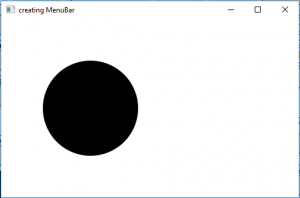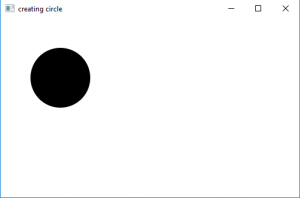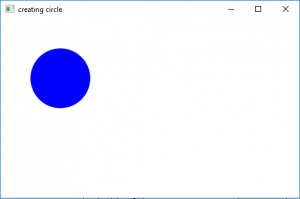# JavaFX | Circle with examples

• Last Updated : 25 Oct, 2019

Circle class is a part of the JavaFX library. Circle class creates a circle with a specified x and y position for the center of the circle, the specified radius for the circle and a specified fill.
The radius and the center of the circles are measured in pixels.

Constructors of the class are:

1. Circle(): creates a empty instance of circle
2. Circle(double r): creates a circle with a specified radius
3. Circle(double X, double Y, double r): creates a circle with given X and y coordinates of the center of the circle, and the radius.
4. Circle(double r, paint f) creates a circle with a specified radius and fill
5. Circle(double X, double Y, double r, Paint f): creates a circle with given X and y coordinates of the center of the circle, and the radius and also a specified fill .

Commonly used methods:

methodexplanation
getCenterX()returns the x coordinate of the center of the circle
getCenterX()returns the y coordinate of the center of the circle
setCenterX(double c)sets the x coordinate of the center of the circle
setCenterY(double c)sets the y coordinate of the center of the circle
setFill(Paint p)sets the fill for the circle

Below programs illustrate the use of the Circle class:

1. Java program to create a circle by passing the coordinates of the center and radius as arguments in constructor: This program creates a Circle indicated by the name circle( the coordinates of the center and the radius is passed as arguments). The Circle will be created inside a scene, which in turn will be hosted inside a stage. The function setTitle() is used to provide title to the stage. Then a Group is created, and the circle is attached. The group is attached to the scene. Finally, the show() method is called to display the final results.

 `// Java program to create circle by passing the``// coordinates of the center and radius``// as arguments in constructor``import` `javafx.application.Application;``import` `javafx.scene.Scene;``import` `javafx.scene.control.Button;``import` `javafx.scene.layout.*;``import` `javafx.event.ActionEvent;``import` `javafx.scene.shape.Circle;``import` `javafx.scene.control.*;``import` `javafx.stage.Stage;`` ` `import` `javafx.scene.Group;``public` `class` `circle_0 ``extends` `Application {`` ` `    ``// launch the application``    ``public` `void` `start(Stage stage)``    ``{``        ``// set title for the stage``        ``stage.setTitle(``"creating circle"``);`` ` `        ``// create a circle``        ``Circle circle = ``new` `Circle(``150``.0f, ``150``.0f, ``80``.f);`` ` `        ``// create a Group``        ``Group group = ``new` `Group(circle);`` ` `        ``// create a scene``        ``Scene scene = ``new` `Scene(group, ``500``, ``300``);`` ` `        ``// set the scene``        ``stage.setScene(scene);`` ` `        ``stage.show();``    ``}`` ` `    ``public` `static` `void` `main(String args[])``    ``{``        ``// launch the application``        ``launch(args);``    ``}``}`

Output:2. Java program to create a circle and using the functions setCenterX, setCenterY and setRadius to set the coordinates of center and radius: This program creates a Circle indicated by the name circle. The coordinates for the center and the radius of the circle is set using setCenterX(), setCenterY(), and setRadius function. The Circle will be created inside a scene, which in turn will be hosted inside a stage. The function setTitle() is used to provide title to the stage. Then a Group is created, and the circle is attached. The group is attached to the scene. Finally, the show() method is called to display the final results.

 `// Java program to create a circle and using ``// the functions setCenterX, setCenterY and setRadius``// to set the coordinates of center and radius``import` `javafx.application.Application;``import` `javafx.scene.Scene;``import` `javafx.scene.control.Button;``import` `javafx.scene.layout.*;``import` `javafx.event.ActionEvent;``import` `javafx.scene.shape.Circle;``import` `javafx.scene.control.*;``import` `javafx.stage.Stage;`` ` `import` `javafx.scene.Group;``public` `class` `circle_1 ``extends` `Application {`` ` `    ``// launch the application``    ``public` `void` `start(Stage stage)``    ``{``        ``// set title for the stage``        ``stage.setTitle(``"creating circle"``);`` ` `        ``// create a circle``        ``Circle circle = ``new` `Circle();`` ` `        ``// set the position of center of the  circle``        ``circle.setCenterX(``100``.0f);``        ``circle.setCenterY(``100``.0f);`` ` `        ``// set Radius of the circle``        ``circle.setRadius(``50``.0f);`` ` `        ``// create a Group``        ``Group group = ``new` `Group(circle);`` ` `        ``// create a scene``        ``Scene scene = ``new` `Scene(group, ``500``, ``300``);`` ` `        ``// set the scene``        ``stage.setScene(scene);`` ` `        ``stage.show();``    ``}`` ` `    ``public` `static` `void` `main(String args[])``    ``{``        ``// launch the application``        ``launch(args);``    ``}``}`

Output:3. Java program to create a circle with specified radius and coordinates of center and also specified fill: This program creates a Circle indicated by the name circle.The coordinates for the center and the radius of the circle is set using setCenterX(), setCenterY(), and setRadius function. .The function set Fill() is used to set the fill of the circle The Circle will be created inside a scene, which in turn will be hosted inside a stage. The function setTitle() is used to provide title to the stage. Then a Group is created, and the circle is attached.The group is attached to the scene. Finally, the show() method is called to display the final results.

 `// Java program to create a circle with specified``// radius and coordinates of center and also specified fill``import` `javafx.application.Application;``import` `javafx.scene.Scene;``import` `javafx.scene.control.Button;``import` `javafx.scene.layout.*;``import` `javafx.scene.paint.Color;``import` `javafx.scene.shape.Circle;``import` `javafx.scene.control.*;``import` `javafx.stage.Stage;`` ` `import` `javafx.scene.Group;``public` `class` `circle_2 ``extends` `Application {`` ` `    ``// launch the application``    ``public` `void` `start(Stage stage)``    ``{``        ``// set title for the stage``        ``stage.setTitle(``"creating circle"``);`` ` `        ``// create a circle``        ``Circle circle = ``new` `Circle();`` ` `        ``// set the position of center of the  circle``        ``circle.setCenterX(``100``.0f);``        ``circle.setCenterY(``100``.0f);`` ` `        ``// set Radius of the circle``        ``circle.setRadius(``50``.0f);`` ` `        ``// set the fill of the circle``        ``circle.setFill(Color.BLUE);`` ` `        ``// create a Group``        ``Group group = ``new` `Group(circle);`` ` `        ``// create a scene``        ``Scene scene = ``new` `Scene(group, ``500``, ``300``);`` ` `        ``// set the scene``        ``stage.setScene(scene);`` ` `        ``stage.show();``    ``}`` ` `    ``public` `static` `void` `main(String args[])``    ``{``        ``// launch the application``        ``launch(args);``    ``}``}`

Output:4. Note : The above programs might not run in an online IDE please use an offline compiler.

My Personal Notes arrow_drop_up